## school closure work for Higher class

We’ve been looking at the photoelectric effect this week.  In this video, Professor Dave reviews some of the points we covered in class.

Click on the picture below to download a simulation to investigate the effect of irradiance on frequency on photocurrent.  You’ll be prompted to install Java if you don’t have it already.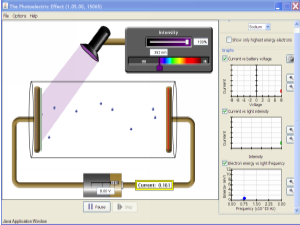Once the animation is running, you can;

• change the metal under investigation (we used zinc in class)
• vary the wavelength of the incident light
• vary the irradiance of the incident light.

Notice that below the theshold frequency you can’t get any photoelectrons, even if you set the light to its brightest setting.

I have attached some notes & questions on the photoelectric effect. Click on the link below to download a copy.

## higher particles and waves revision

Remember that your unit assessment for P&W will take place at the end of this week.  The attached notes might be helpful during your revision.

## particle accelerators

An electric field can be used to accelerate charged particles.

Conservation of energy tells us that

work done by the electric field = change in the particle’s kinetic energy

The speed of the particle can be determined if its charge and the accelerating voltage (potential difference) are known.  The notes attached to the end of this post will show how to perform the calculation.

These short video clips show how to draw electric field lines for point charges and parallel plates, with example calculations for the work done by electric fields and the final speed of charged particles in electric fields.

## quarks, leptons and antimatter

At the end of Our Dynamic Universe, we considered big things like stars, galaxies and the Universe itself.  Now the Particles and Waves unit brings us to particles so small we need groups of them just to make a single atom.  Is there a connection?

Why do we study particles? from mr mackenzie on Vimeo.

### The Standard Model

An elementary (or fundamental) particle is a particle that is not built from other, smaller particles.  Until the start of the 20th century, scientists had believed that atoms were elementary particles.  However, the discovery of the electron (J.J. Thompson), proton (Rutherford), and neutron (Chadwick), together with Rutherford’s evidence for a heavy, positively charged nucleus at the centre of the atom suggested the atom was not an elementary particle after all.

Brian Cox explains in this video clip…

To go further, we have to introduce some particle physics vocabulary.

These new elementary particles are part of our Standard Model of how the building blocks of the universe interact with one another.  The particles that form “matter” are called fermions, after Enrico Fermi  (Fermi has an incredibly long list of things named after him).  The fermions are divided into two groups; quarks and leptons, as shown in the diagram below.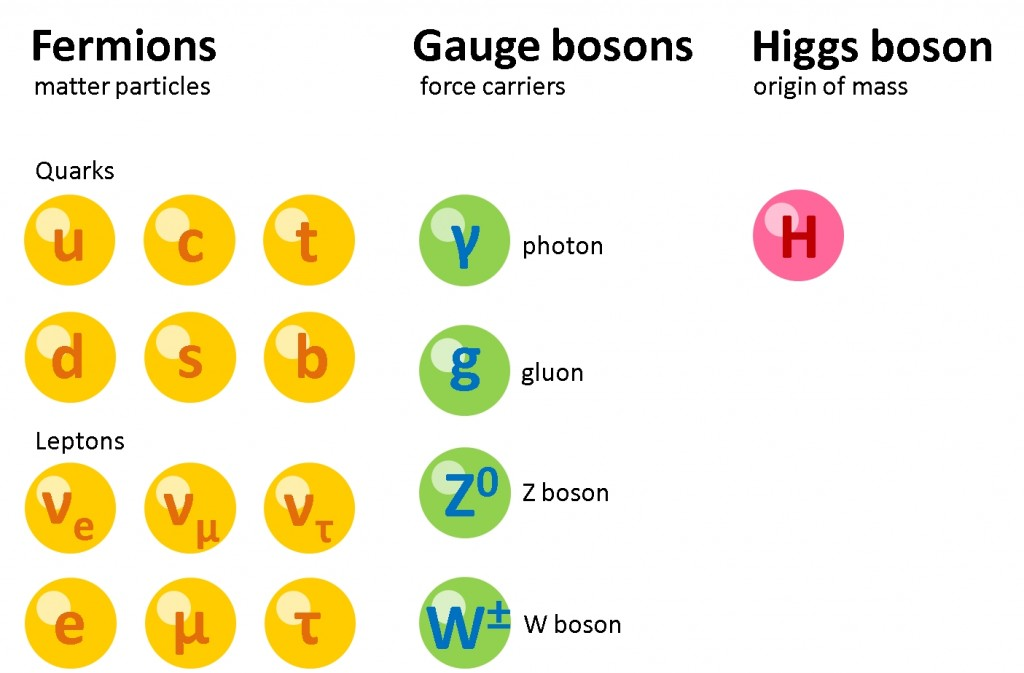## refraction, critical angle and total internal reflection

We met refraction during the National 5 course.  At Higher level, we are interested in the relationship between the angles of incidence θi and refraction θr.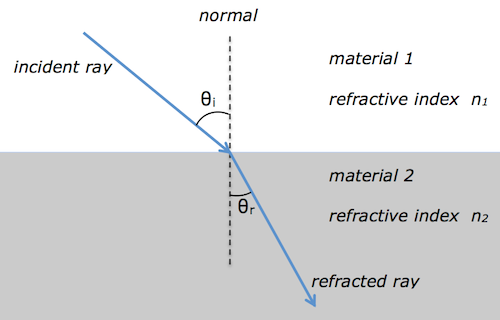Snell’s law tells us that$n_1\sin \theta_i = n_2 \sin \theta_r$

Usually material 1 is air, and so$n_1 = 1$.  This simplifies Snell’s law to$\sin \theta_i = n \sin \theta_r$

where n is the absolute refractive index of material 2.  Since the refractive index is equal to the ratio of the ray’s speed v in materials 1 & 2 and also equal to the ratio of the wave’s wavelength λ in materials 1 & 2, we can show that$n = \displaystyle {{{\sin \theta_i} \over {\sin \theta_r}}} = \displaystyle {v_1 \over v_2} = \displaystyle {\lambda_1 \over \lambda_2}$

### total internal reflection

total internal reflection from mr mackenzie on Vimeo.
The critical angle$\theta_c$ and refractive index n are related by$\sin \theta_c = \displaystyle {1 \over n }$

Here are some applications of total internal reflection here.  You can test your knowledge of refraction with this interactive simulation.

I have attached a pdf with some notes and questions on refraction, total internal reflection and critical angle.

## diffraction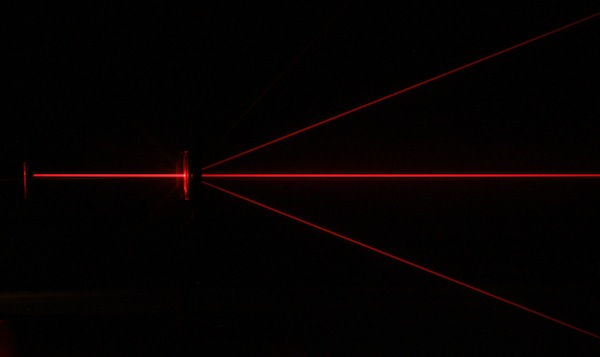###### red laser beam passing through a diffraction grating. image: en.academic.ru

Diffraction is a test for wave behaviour.  When a ray of light passes through a diffraction grating, the energy of the incident beam is split into a series of interference fringes.  Constructive interference is occurring at each location where a fringe (or spot) is observed because the rays are in phase when they arrive at these points.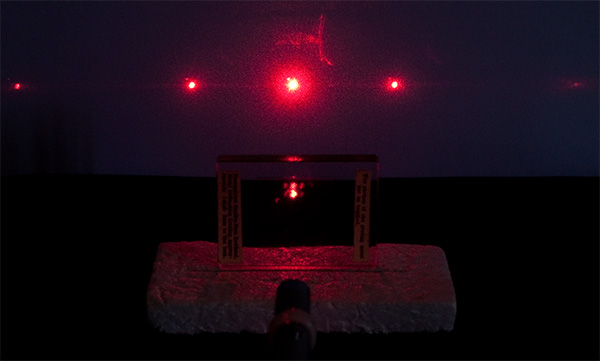###### image: microscopy uk

Find out about diffraction gratings here and here.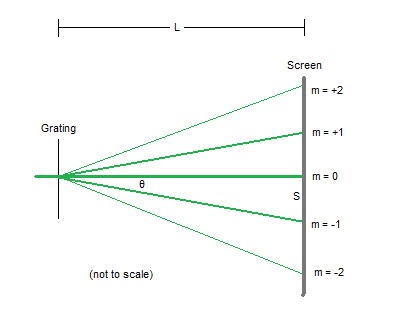###### image: laserpointerforums.com

We can measure the relative positions of the fringes in a diffraction pattern to determine the wavelength of the light used.  The diffraction grating equation is$m \lambda = d \sin \theta$

where

• m is the diffracted order  –  some resources may use n instead of m
• λ is the wavelength
• d is the line spacing.

Here is an infrared diffraction experiment you can try at home to calculate the wavelength of the infrared LED in a remote control.

I’ve attached a set of pdf notes and questions on diffraction.  These notes use n rather than m for the diffracted order.

## the photoelectric effect

We learned about the photoelectric effect this week.  This video has a similar demonstration to the gold leaf electroscope experiment I showed you in class and includes an explanation of the process.

Click on the picture below to download the simulation we used to investigate the effect of irradiance on frequency on photocurrent.  You’ll be prompted to install Java if you don’t have it already.Once the animation is running, you can;

• change the metal under investigation (we used zinc in class)
• vary the wavelength of the incident light
• vary the irradiance of the incident light.

Notice that below the threshold frequency you can’t get any photoelectrons, even if you set the light to its brightest setting.

I have attached some notes & questions on the photoelectric effect. Click on the link below to download a copy.

## introduction to particle physics

There’s a nice guide to particle physics on The Particle Adventure web site.  The site has it’s own free apps for android & apple devices that are worth installing.

Here’s Prof Cox with a two part summary of particle physics.

and a short video with just the quarks

The video below was made before the Higgs boson was confirmed, so please bear that in mind.  It’s still a nice video though.

Learn about the world’s largest neutrino detector in Antarctica.